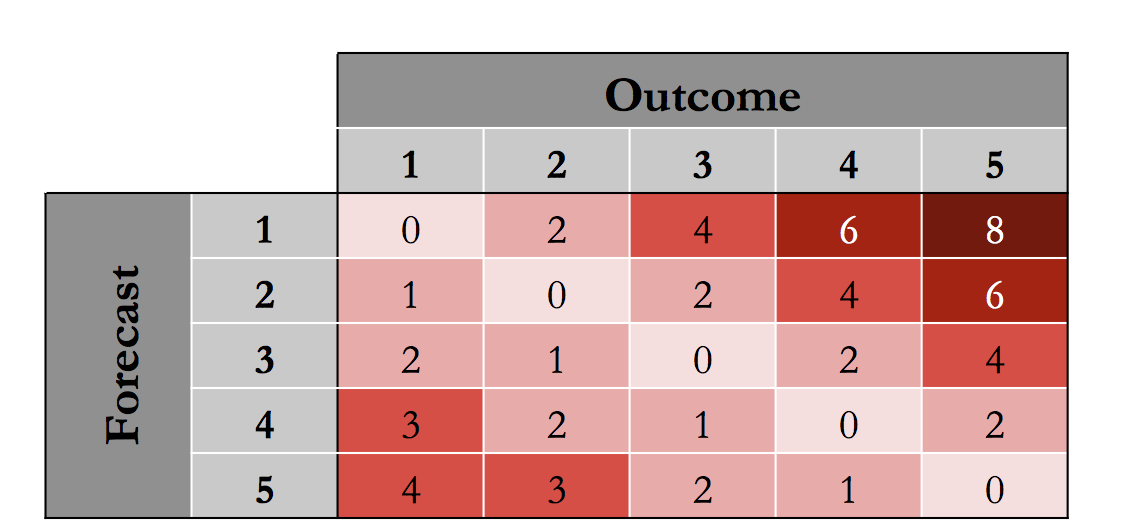# 4.3 Keeping an Eye on Healthcare Costs: The D2Hawkeye Story

## Quick Question

The image below shows the penalty error matrix that we discussed in the previous video.We can interpret this matrix as follows. Suppose the actual outcome for an observation is 3, and we predict 2. We find 3 on the top of the matrix, and go down to the second row (since we forecasted 2). The penalty error for this mistake is 2. If for another observation we predict (forecast) 4, but the actual outcome is 1, that is a penalty error of 3.

What is the worst mistake we can make, according to the penalty error matrix?

We predict 5 (very high cost), but the actual outcome is 1 (very low cost).  close

We predict 1 (very low cost), but the actual outcome is 5 (very high cost).  check

Explanation The highest cost is 8, which occurs when the forecast is 1 (very low cost), but the actual cost is 5 (very high cost). It would be much worse for us to ignore an actual high cost observation than to accidentally predict high cost for someone who turns out to be low cost

What are the “best” types of mistakes we can make, according to the penalty error matrix?

Mistakes where we predict one cost bucket HIGHER than the actual outcome.  check

Mistakes where we predict one cost bucket LOWER than the actual outcome.  close

Explanation We are happier with mistakes where we predict one cost bucket higher than the actual outcome, since this just means we are being a little overly cautious.

#### Learning Resource Types

theaters Lecture Videos
notes Lecture Notes
assignment_turned_in Problem Sets with Solutions